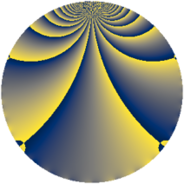# Properties

 Label 1694.2.oLevel $1694$ Weight $2$ Character orbit 1694.o Rep. character $\chi_{1694}(153,\cdot)$ Character field $\Q(\zeta_{22})$ Dimension $880$ Sturm bound $528$

# Related objects

## Defining parameters

 Level: $$N$$ $$=$$ $$1694 = 2 \cdot 7 \cdot 11^{2}$$ Weight: $$k$$ $$=$$ $$2$$ Character orbit: $$[\chi]$$ $$=$$ 1694.o (of order $$22$$ and degree $$10$$) Character conductor: $$\operatorname{cond}(\chi)$$ $$=$$ $$847$$ Character field: $$\Q(\zeta_{22})$$ Sturm bound: $$528$$

## Dimensions

The following table gives the dimensions of various subspaces of $$M_{2}(1694, [\chi])$$.

Total New Old
Modular forms 2680 880 1800
Cusp forms 2600 880 1720
Eisenstein series 80 0 80

## Trace form

 $$880q + 88q^{4} - 872q^{9} + O(q^{10})$$ $$880q + 88q^{4} - 872q^{9} + 40q^{11} + 14q^{14} - 28q^{15} - 88q^{16} - 40q^{22} - 32q^{23} + 80q^{25} - 96q^{36} - 116q^{37} + 20q^{42} + 4q^{44} + 56q^{49} - 44q^{51} + 4q^{53} + 8q^{56} + 32q^{58} - 16q^{60} - 110q^{63} + 88q^{64} - 72q^{67} - 32q^{70} - 16q^{71} + 40q^{77} - 32q^{78} + 176q^{79} + 800q^{81} - 220q^{85} - 24q^{86} - 4q^{88} - 66q^{91} - 144q^{92} + 100q^{93} - 176q^{95} - 88q^{98} - 128q^{99} + O(q^{100})$$

## Decomposition of $$S_{2}^{\mathrm{new}}(1694, [\chi])$$ into newform subspaces

The newforms in this space have not yet been added to the LMFDB.

## Decomposition of $$S_{2}^{\mathrm{old}}(1694, [\chi])$$ into lower level spaces

$$S_{2}^{\mathrm{old}}(1694, [\chi]) \cong$$ $$S_{2}^{\mathrm{new}}(847, [\chi])$$$$^{\oplus 2}$$